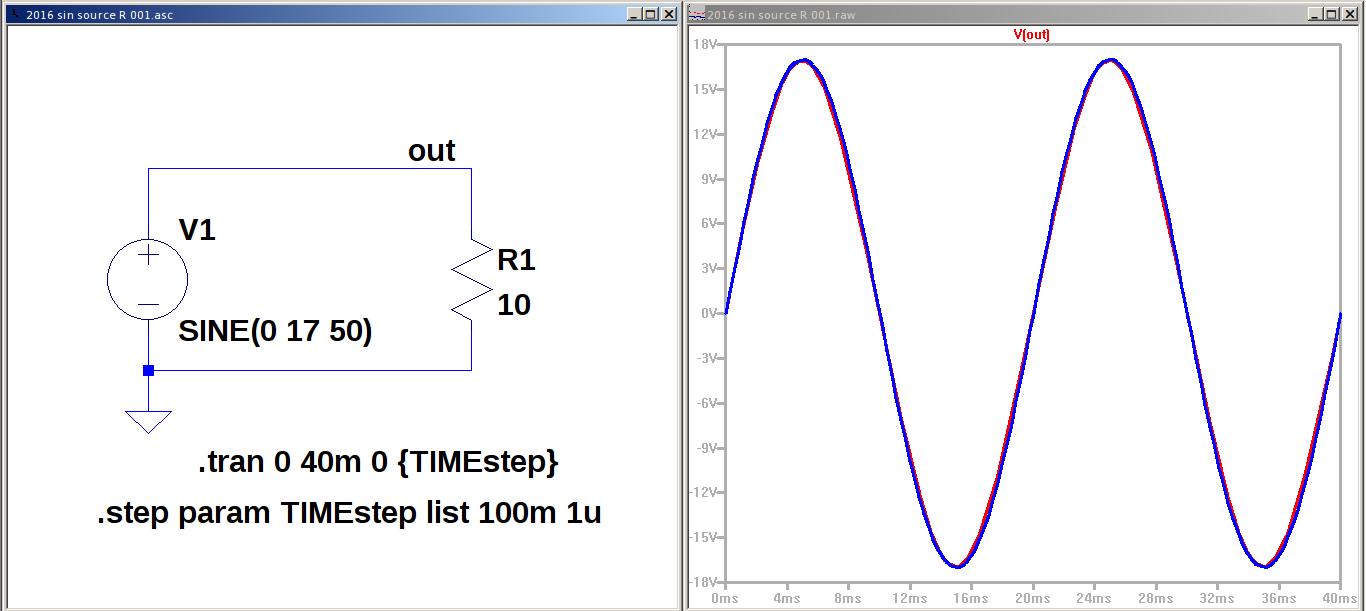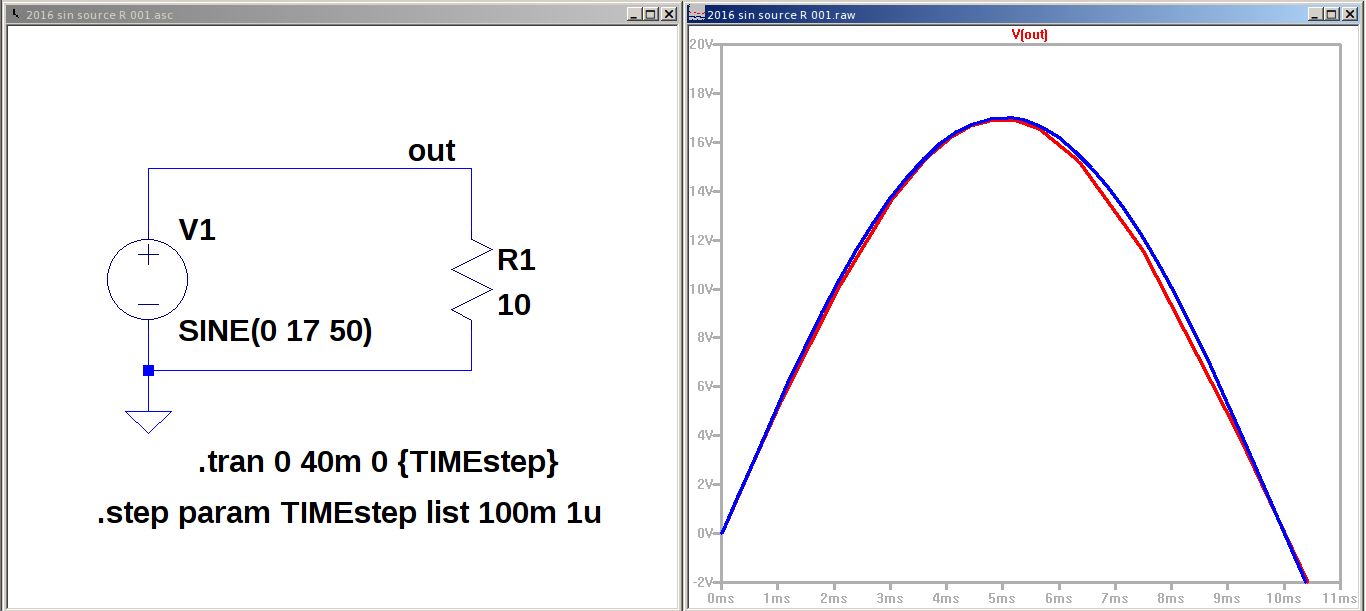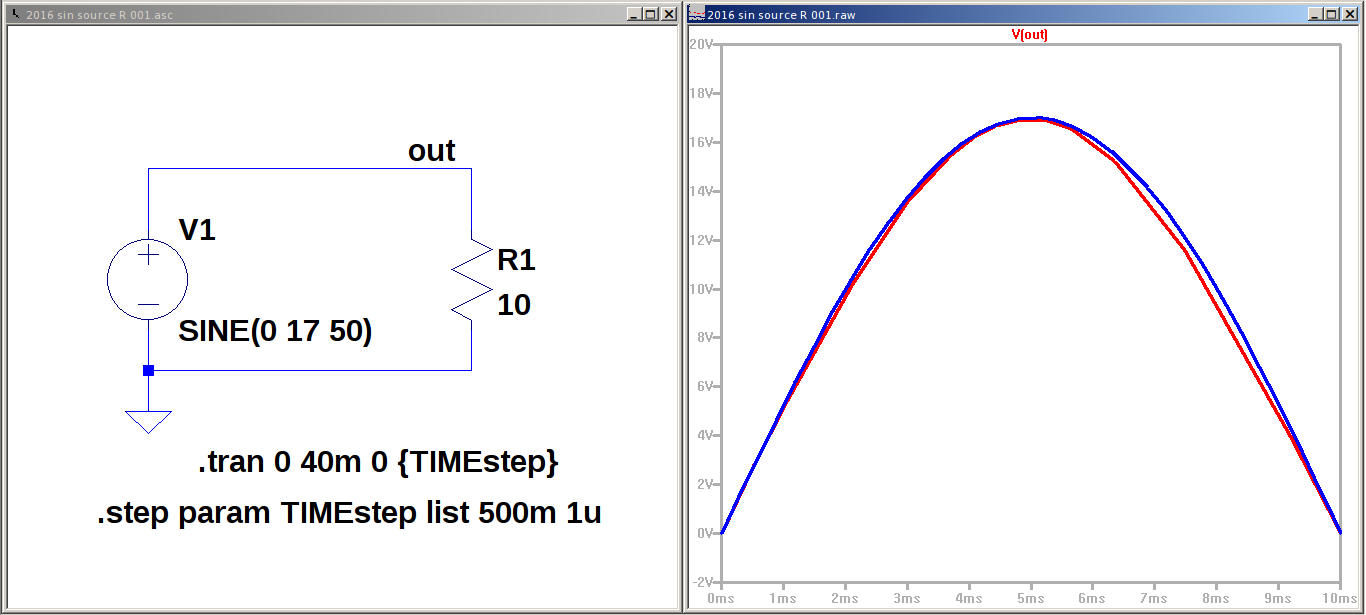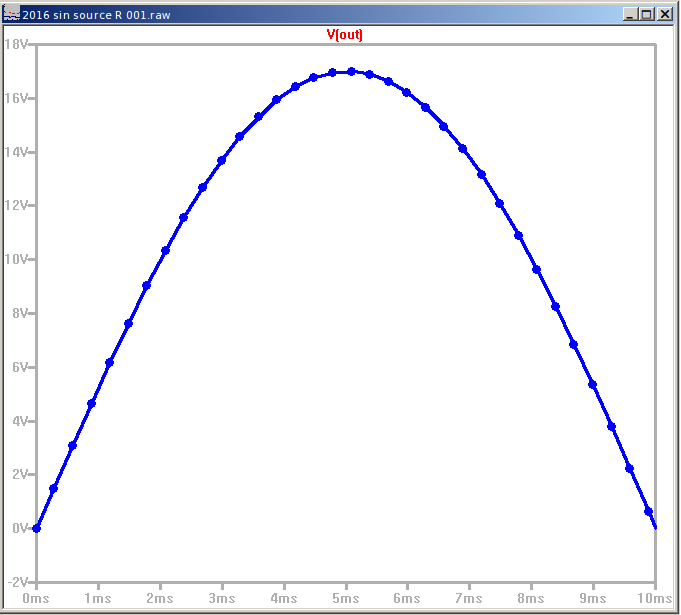# Pengaruh resolusi / ketelitian pada simulasi dan perhitungan.

Mari memulai dengan suatu contoh yang sangat sederhana. Berapakan empat ditambah dengan tiga di dalam perhitungan basis sepuluh?

Sayangnya tidak semua perhitungan semudah dan setepat sebagaimana pada Gambar 1. Ada perbedaan pada hasil perhitungan (dan karenanya) hasil simulasi yang perlu diperhatikan sehingga tidak akan menjadi sumber kebingungan dan bahkan keraguan terhadap landasan teoritis yang sebenarnya tidak memiliki alasan yang cukup kuat. Terutama dalam engineering technology yang titik beratnya adalah untuk memanfaatkan temuan-temuan dalam sains dan teknologi.Gambar 2.Gambar 3.Gambar 4.Gambar 5.Gambar 6.Gambar 7.

https://en.wikipedia.org/wiki/Round-off_error

A round-off error, also called rounding error, is the difference between the calculated approximation of a number and its exact mathematical value due to rounding. This is a form of quantization error. One of the goals of numerical analysis is to estimate errors in calculations, including round-off error, when using approximation equations and/or algorithms, especially when using finitely many digits to represent real numbers (which in theory have infinitely many digits).

When a sequence of calculations subject to rounding error is made, errors may accumulate, sometimes dominating the calculation. In ill-conditioned problems, significant error may accumulate.

The error introduced by attempting to represent a number using a finite string of digits is a form of round-off error called representation error. Here are some examples of representation error in decimal representations:Gambar 9.

How to correct rounding errors in floating-point arithmetic

Many combinations of arithmetic operations on floating-point numbers in Microsoft Excel and Microsoft Works may produce results that appear to be incorrect by very small amounts. For example, the equation
=1*(.5-.4-.1)
may be evaluated to the quantity (-2.78E-17), or -0.0000000000000000278 instead of 0.

The IEEE 754 standard is a method of storing floating-point numbers in a compact way that is easy to manipulate. This standard is used by Intel coprocessors and most PC-based programs that implement floating-point math.

IEEE 754 specifies that numbers be stored in binary format to reduce storage requirements and allow the built-in binary arithmetic instructions that are available on all microprocessors to process the data in a relatively rapid fashion. However, some numbers that are simple, nonrepeating decimal numbers are converted into repeating binary numbers that cannot be stored with perfect accuracy.

For example, the number 1/10 can be represented in a decimal number system with a simple decimal:
.1
However, the same number in binary format becomes the repeating binary decimal:
.0001100011000111000111 (and so on)
This number cannot be represented in a finite amount of space. Therefore, this number is rounded down by approximately -2.78E-17 when it is stored.

If several arithmetic operations are performed to obtain a given result, these rounding errors may be cumulative.

Rounding Error
Jeffrey L. Popyack, June 2000

Rounding (roundoff) error is a phenomenon of digital computing resulting from the computer’s inability to represent some numbers exactly. Specifically, a computer is able to represent exactly only integers in a certain range, depending on the word size used for integers. Certain floating-point numbers may also be represented exactly, depending on the representation scheme in use on the computer in question and the word size used for floating-point numbers. Certain floating-point numbers cannot be represented exactly, regardless of the word size used.

Errors due to rounding have long been the bane of analysts trying to solve equations and systems. Such errors may be introduced in many ways, for instance:

+ inexact representation of a constant

+ integer overflow resulting from a calculation with a result too large for the word size

+ integer overflow resulting from a calculation with a result too large for the number of bits used to represent the mantissa of a floating-point number

+ accumulated error resulting from repeated use of numbers stored inexactly

Summary

Rounding error is a natural consequence of the representation scheme used for integers and floating-point numbers in digital computers. Rounding can produce highly inaccurate results as errors get propagated through repeated operations using inaccurate numbers. Proper handling of rounding error may involve a combination of approaches such as use of high-precision data types and revised calculations and algorithms. Mathematical analysis can be used to estimate the actual error in calculations.

https://en.wikipedia.org/wiki/Rounding

Rounding a numerical value means replacing it by another value that is approximately equal but has a shorter, simpler, or more explicit representation; for example, replacing £23.4476 with £23.45, or the fraction 312/937 with 1/3, or the expression √2 with 1.414.

Rounding is often done to obtain a value that is easier to report and communicate than the original. Rounding can also be important to avoid misleadingly precise reporting of a computed number, measurement or estimate; for example, a quantity that was computed as 123,456 but is known to be accurate only to within a few hundred units is better stated as “about 123,500”.

On the other hand, rounding of exact numbers will introduce some round-off error in the reported result. Rounding is almost unavoidable when reporting many computations — especially when dividing two numbers in integer or fixed-point arithmetic; when computing mathematical functions such as square roots, logarithms, and sines; or when using a floating point representation with a fixed number of significant digits. In a sequence of calculations, these rounding errors generally accumulate, and in certain ill-conditioned cases they may make the result meaningless.

Accurate rounding of transcendental mathematical functions is difficult because the number of extra digits that need to be calculated to resolve whether to round up or down cannot be known in advance. This problem is known as “the table-maker’s dilemma”.

Rounding has many similarities to the quantization that occurs when physical quantities must be encoded by numbers or digital signals.

A wavy equals sign (≈) is sometimes used to indicate rounding of exact numbers. For example: 9.98 ≈ 10.

https://en.wikipedia.org/wiki/Significant_figures

The significant figures of a number are digits that carry meaning contributing to its measurement resolution. This includes all digits except: